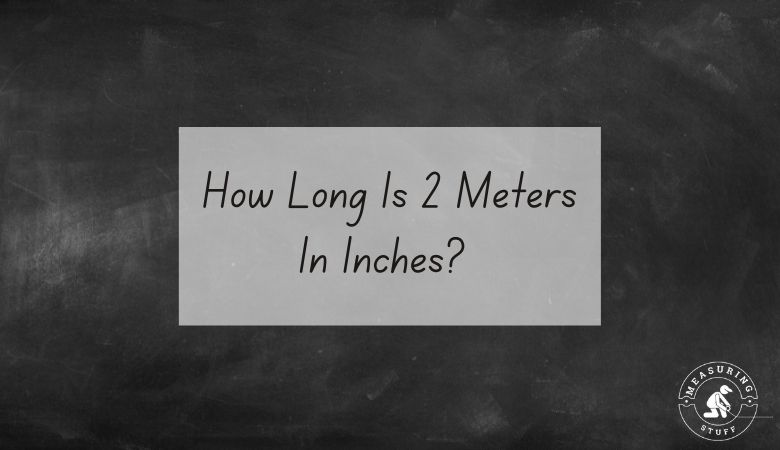# How Long Is 2 Meters In Inches?

Last updated on September 4th, 2022 at 09:35 pm

2 meters equals 78.740 inches.

To convert 2 meters into inches, you need to multiply by the conversion factor of 39.37 as there are that many inches in 1 meter.

For example, use the following formula and multiply the number of meters by 39.37 to get the answer in inches.

2 meters x 39.37 (conversion factor) = 78.740 inches.

This means that there are 78.740 inches in 2 meters.

If you want to reverse the question and figure out how many meters can be made from a certain number of inches, you would divide the number of inches by 39.37.

For example, if you have 78.740 inches, you can divide it by 39.37 to get 2 meters.

This means that there are 2 meters in 78.740 inches.

If you don’t feel like doing the math, use our online conversion calculator below to convert different units of measurements.

## Meters into inches conversion calculator

Use the following calculator to easily convert meters into inches. Simply type in the desired value and select meters in the convert from box and inches in the convert to box. The calculator will instantly do the math for you.

Use the following table to convert meters into inches.

## Meters into inches conversion table

You can also convert 2 meters into other units of measurements.

• 2 meters = 6.562 feet
• 2 meters = 200 centimeters
• 2 meters = 2000 millimeters
CHECK OUT  How Heavy Is 4 Tons? (11 Interesting Examples)

Other articles on our site using meters and inches include:

## Meters measurement

A Meter is part of the metric system of measurement which was created in France to have a way to measure length, mass, and volume. 1 meter is equal to 3 feet 3 inches in length.

## Inches measurement

An inch is a unit of linear measure equal to one-twelfth of a foot (2.54 cm).

Inch or inches is a length unit of measurement. It is used in the USA as a customary and Imperial unit of length.

Many people will abbreviate the word inch as in. For example, 1 inch may be written as 1 in. It can also be denoted by using the double prime symbol , for example, 1 inch can be written as 1″.

1 inch is also equal to 1⁄36 yard or 1⁄12 foot.